Home > ACC7 > Chapter cc38 > Lesson cc38.2.3 > Problem8-92

8-92.
1. For each equation below, solve for x. Sometimes the easiest strategy is to use mental math. Homework Help ✎

1.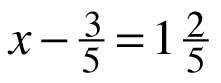2. 5.2 + x = 10.95

3. 2x − 3.25 = 7.15

4.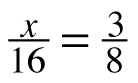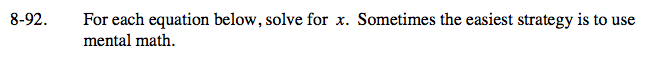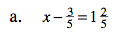$x-\frac{3}{5} = \frac{7}{5}$

Can you use mental math to solve this equation?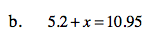x = 5.75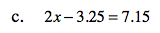x = 5.2
Be sure to know how to get this answer using mental math. Recognize that 3.25 + 7.15 equals an even decimal, 10.4.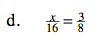8(2) = 16
What must x be?